×

Search anything:

# Topological Sorting using Depth First Search (DFS)

#### Algorithms Graph Algorithms topological sort depth first searchGet this book -> Problems on Array: For Interviews and Competitive Programming

Reading time: 25 minutes | Coding time: 12 minutes

Topological Sorting for Directed Acyclic Graph (DAG) is a linear ordering of vertices such that for every directed edge uv, vertex u comes before v in the ordering.A topological ordering is possible if and only if the graph has no directed cycles, that is, if it is a directed acyclic graph (DAG). Any DAG has at least one topological ordering.

In this article, we will explore how we can implement Topological sorting using Depth First Search.

### Algorithm using Depth First Search

Here we are implementing topological sort using Depth First Search.

• Step 1: Create a temporary stack.
• Step 2: Recursively call topological sorting for all its adjacent vertices, then push it to the stack (when all adjacent vertices are on stack). Note this step is same as Depth First Search in a recursive way.
• Step 3: Atlast, print contents of stack.

Note: A vertex is pushed to stack only when all of its adjacent vertices (and their adjacent vertices and so on) are already in stack.

In another way, you can think of this as Implementing Depth First Search to process all nodes in a backtracking way

### Pseudocode

The pseudocode of topological sort is:

• Step 1: Create the graph by calling addEdge(a,b).
• Step 2: Call the topologicalSort( )
• Step 2.1: Create a stack and a boolean array named as visited[ ];
• Step 2.2: Mark all the vertices as not visited i.e. initialize visited[ ] with 'false' value.
• Step 2.3: Call the recursive helper function topologicalSortUtil() to store Topological Sort starting from all vertices one by one.
• Step 3: def topologicalSortUtil(int v, bool visited[],stack<int> &Stack):
• Step 3.1: Mark the current node as visited.
• Step 3.2: Recur for all the vertices adjacent to this vertex.
• Step 3.3: Push current vertex to stack which stores result.
• Step 4: Atlast after return from the utility function, print contents of stack.

### Example

Consider the following graph: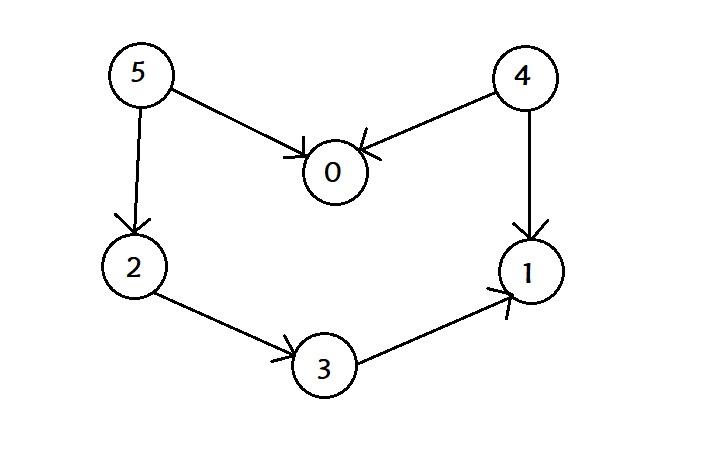Following is the adjacency list of the given graph: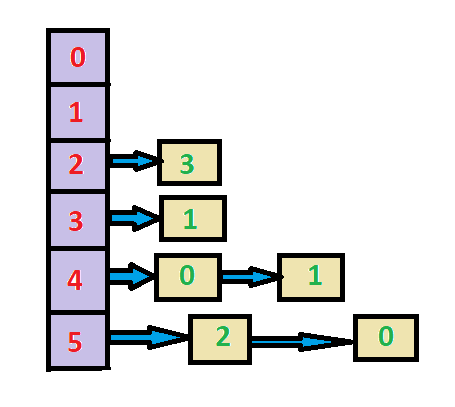When topological() is called: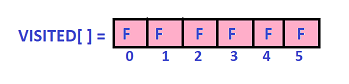Stepwise demonstration of the stack after each iteration of the loop(topologicalSort()):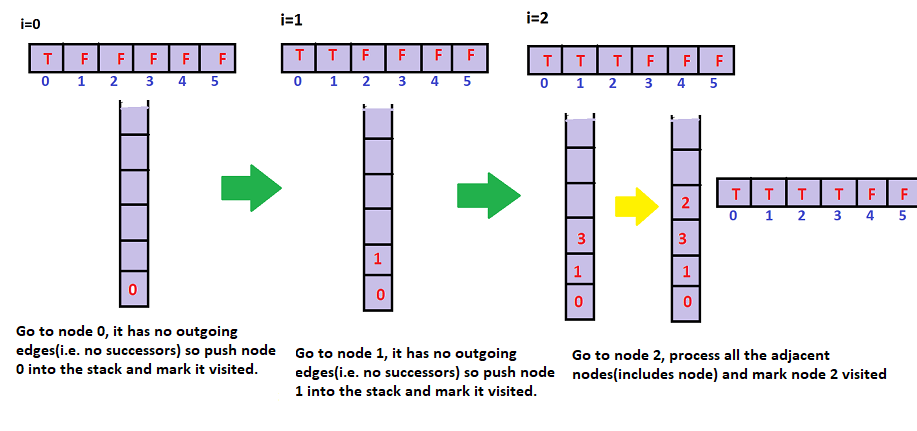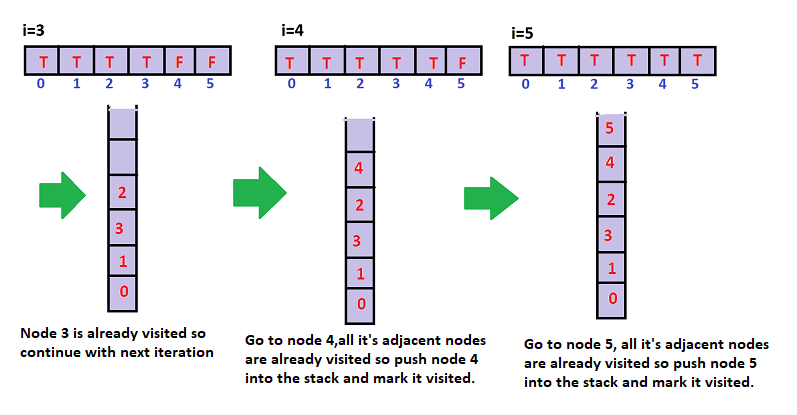The contents of the stack are: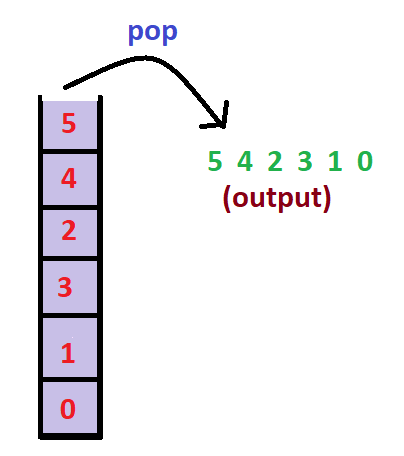So the topological sorting of the above graph is “5 4 2 3 1 0”. There can be more than one topological sorting for a graph. For example, another topological sorting of the above graph is “4 5 2 3 1 0”. Both of them are correct!

### Complexity

Worst case time complexity:`Θ(|V|+|E|)`
Average case time complexity:`Θ(|V|+|E|)`
Best case time complexity:`Θ(|V|+|E|)`
Space complexity:`Θ(|V|)`

The above algorithm is DFS with an extra stack. So time complexity is same as DFS which is O(V+E)

# Implementation

``````// A C++ program to print topological sorting of a DAG
#include <iostream>
#include <list>
#include <stack>
using namespace std;

class Graph
{
int V;

void topologicalSortUtil(int v, bool visited[], stack<int> &Stack);
public:
Graph(int V);

void topologicalSort();
};

Graph::Graph(int V)
{
this->V = V;
}

{
}

void Graph::topologicalSortUtil(int v, bool visited[],
stack<int> &Stack)
{

visited[v] = true;

list<int>::iterator i;
if (!visited[*i])
topologicalSortUtil(*i, visited, Stack);

Stack.push(v);
}

void Graph::topologicalSort()
{
stack<int> Stack;

// Mark all the vertices as not visited
bool *visited = new bool[V];
for (int i = 0; i < V; i++)
visited[i] = false;

for (int i = 0; i < V; i++)
if (visited[i] == false)
topologicalSortUtil(i, visited, Stack);

while (Stack.empty() == false)
{
cout << Stack.top() << " ";
Stack.pop();
}
}

int main()
{

int n,v1,v2;
cin>>n;
Graph g(n);
for(int i=1;i<=n;i++)
{	cin>>v1>>v2;

cout << " Topological Sort of the given graph \n";
g.topologicalSort();

return 0;
}
``````

### Applications

Topological Sorting is mainly used for:

• scheduling jobs from the given dependencies among jobs.

• In computer science, applications of this type arise in:

• instruction scheduling
• ordering of formula cell evaluation when recomputing formula values in spreadsheets
• logic synthesis
• determining the order of compilation tasks to perform in makefiles
• data serialization
• resolving symbol dependencies in linkers.

## Question

#### Topological sort is equivalent to which of the traversals in trees?

Pre-order traversal
Post-order traversal
In-order traversal
Level-order traversall
In pre-order traversal of trees, we process the root first and then child from left to right.#### Saranya Jena

Student at Kalinga Institute of Industrial Technology, Bhubaneswar

Topological Sorting using Depth First Search (DFS)